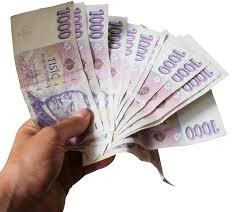# Two-fifths 63014

We divided a certain amount of money between three people so that the first got two-fifths of what the second and third combined. The second got twice as much as the first. If the third received €750 less than the first, how much did each receive?

a =  1500
b =  3000
c =  750

### Step-by-step explanation:Did you find an error or inaccuracy? Feel free to write us. Thank you!

Tips for related online calculators
Need help calculating sum, simplifying, or multiplying fractions? Try our fraction calculator.
Do you have a system of equations and looking for calculator system of linear equations?